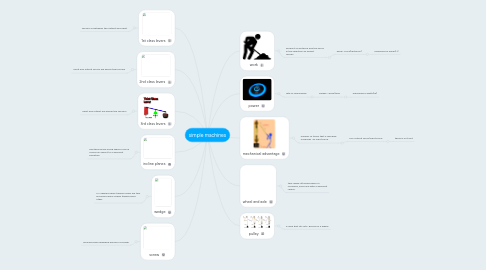# simple machinesRiley Suttles
Get Started. It's Freesimple machines## 1. work

### 1.1. product of distance and the force in the direction an object moves.

1.1.1. work= force(distance)

1.1.1.1. measured in joules(J)

## 2. power

### 2.1. rate of doing work

2.1.1. power= work/time

2.1.1.1. measured in watts(w)

### 3.1. number of times that a machine increases an input force.

3.1.1. MA=output force/input force

3.1.1.1. there is not unit